# CBSE Class 10 Mathematics Application of Trigonometry MCQs

## MCQ for Class 10 Mathematics Application of Trigonometry

Class 10 Mathematics students should refer to the following multiple-choice questions with answers for Application of Trigonometry in standard 10. These MCQ questions with answers for Grade 10 Mathematics will come in exams and help you to score good marks

### Application of Trigonometry MCQ Questions Class 10 Mathematics with Answers

Question. The angle of elevation of the top of a tower from a point on the ground, which is 20m away from the foot of the tower is 600. Find the height of the tower.
(a) 10 √3 m
(b) 30 √3 m
(c) 20 √3 m
(d) none of these

Question. The height of a tower is 10m. What is the length of its shadow when Sun’s altitude is 450?
(a) 10 m
(b) 30 m
(c) 20 m
(d) none of these

Question. The angle of elevation of a ladder leaning against a wall is 600 and the foot of the ladder is 9.5 m away from the wall. Find the length of the ladder.
(a) 10 m
(b) 19 m
(c) 20 m
(d) none of these

Question. If the ratio of the height of a tower and the length of its shadow is √3 : 1, what is the angle of elevation of the Sun?
(a) 300
(b) 600
(c) 450
(d) none of these

Question. What is the angle of elevation of the Sun when the length of the shadow of a vertical pole is equal to its height?
(a) 300
(b) 600
(c) 450
(d) none of these

Question. From a point on the ground, 20 m away from the foot of a vertical tower, the angle of elevation of the top of the tower is 600, what is the height of the tower?
(a) 10 √3 m
(b) 30 √3 m
(c) 20 √3 m
(d) none of these

Question. If the angles of elevation of the top of a tower from two points at a distance of 4 m and 9 m from the base of the tower and in the same straight line with it are complementary, find the height of the tower.
(a) 10 m
(b) 6 m
(c) 8 m
(d) none of these

Question. In the below fig. what are the angles of depression from the observing positions D and E of the object A?(a) 300, 450
(b) 600 , 450
(c) 450, 600
(d) none of these

Question. The ratio of the length of a rod and its shadow is 1: 3 . The angle of elevation of the sun is
(a) 300
(b) 600
(c) 450
(d) none of these

Question. If the angle of elevation of a tower from a distance of 100m from its foot is 600, then the height of the tower is
(a) 100 √3 m
(b) 200/ √3 m
(c) 50 √3 m
(d) 100/√3 m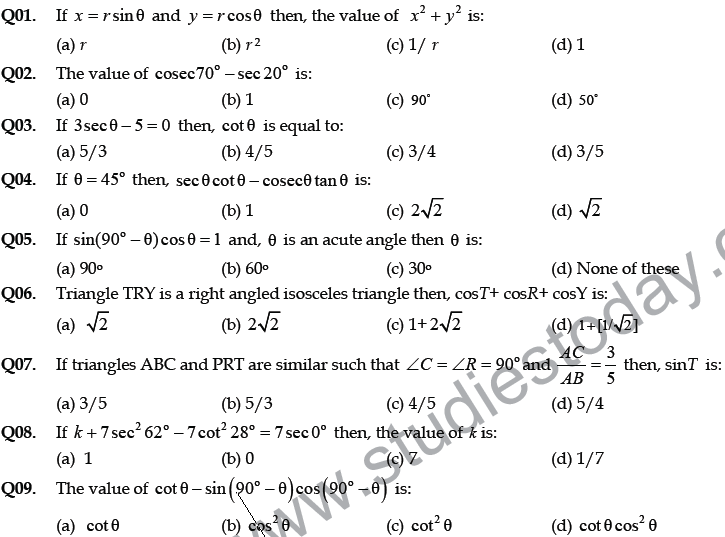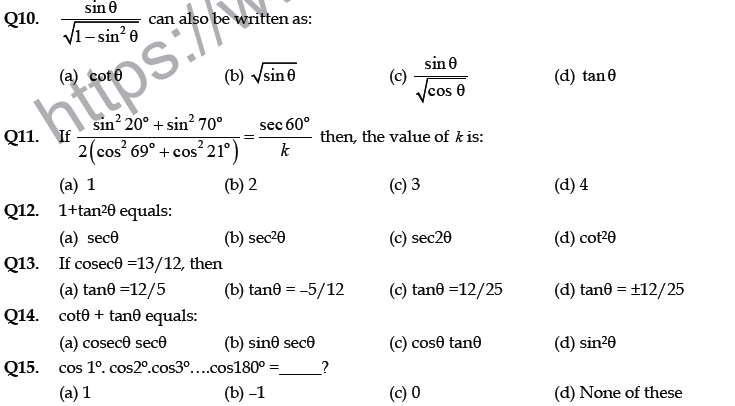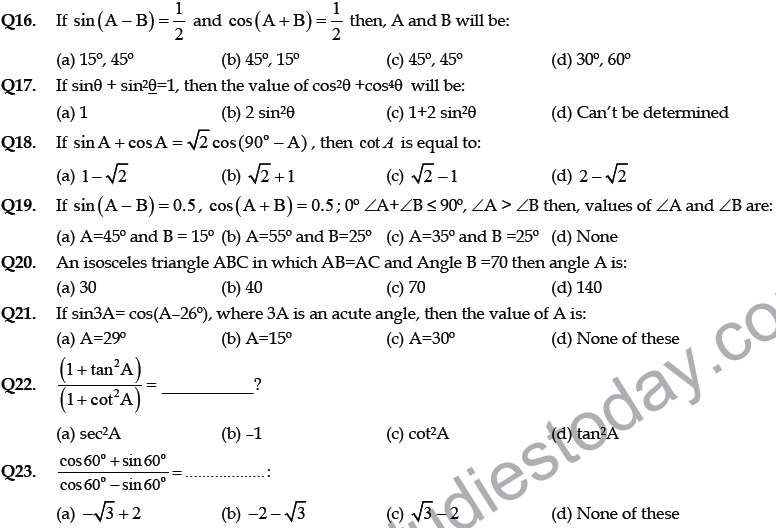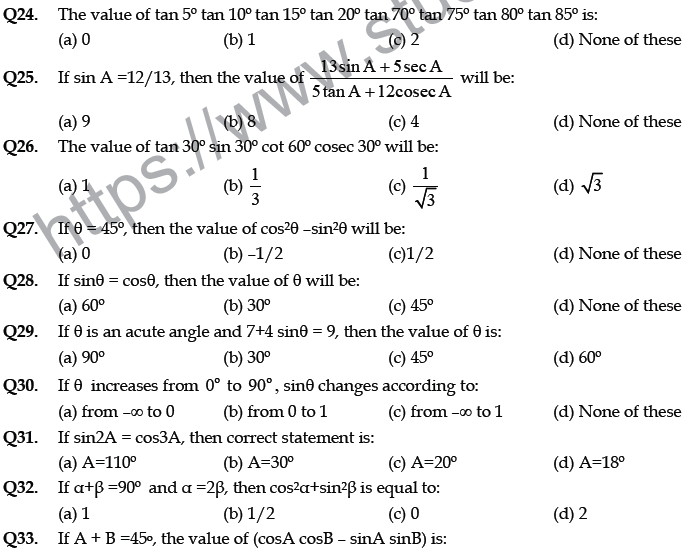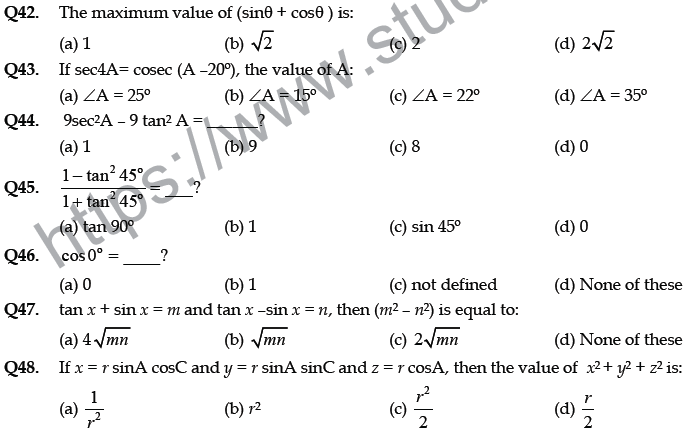Q01. b Q02. a Q03. c Q04. a Q05. d Q06. a Q07. a

Q08. b Q09. d Q10. d Q11. d Q12. b Q13. a Q14. a

Q15. c Q16. b Q17. a Q18. c Q19. a Q20. b Q21. a

Q22. d Q23. b Q24. b Q25. d Q26. b Q27. a Q28. c

Q29. b Q30. b Q31. d Q32. b Q33. c Q34. c Q35. b

Q36. b Q37. d Q38. a Q39. c Q40. c Q41. c Q42. b

Q43. c Q44. b Q45. b Q46. b Q47. a Q48. b

Question : If cos A =4/5 , then the value of tan A is :
(a) 3/5
(b) 3/4
(c) 4/3
(d) 5/3

Question : If sin θ = a/b , then cos θ is equal to :
(a)  a /√(b2 – a2)
(b)  b/a
(c)  √(b2–a2) /b
(d)  a/√(b2 – a2)

Question : How many values of θ satisfies the following : sin2 θ = 1– 1/sec2 θ
(a) 0
(b) 1
(c) more then 1 but less then 20
(d) infinite

Question : If 4 tan θ = 3, then ( 4sin cos /4sin – cos ) is equal to :
(a) 2/3
(b) 1/3
(c) 1/2
(d) 3/4

Question : 1 sin2 45º /1 – sin2 45º is equal to :
(a) cos 60°
(b) sin2 60°
(c) tan2 30°
(d) sin 30°

Question : The value of (sin 30° cos 60° + cos 30° sin 60°) is :
(a) sin 90°
(b) cos 90°
(c) sin 0°
(d) cos 30°

Question : sinθ / 1+cosθ is equal to :
(a) 1+ cosθ /sinθ
(b) 1 – cotθ/sinθ
(c) 1 – cosθ/sinθ
(d) 1 – sinθ /cosθ

Question : If x = a cos α and y = b sin α, then b2x2 + a2y2 is equal to :
(a) a2b2
(b) ab
(c) a4b4
(d) a2 + b2

Question : If cos 9α= sin α and 9α< 90°, then the value of tan 5α is :
(a) 0
(b) 1
(c) 3
(d) cannot be determined

Question : In the given figure, ∠ACB = 90°, ∠BDC = 90°, CD = 4 cm, BD = 3 cm, AC = 12 cm, cos A – sin A is equal to :
(a) 5/12
(b) 5/13
(c) 7/12
(d) 7/13Question : At a certain instant, the altitude of the sun is 60º. At that instant, the length of the shadow of a vertical tower is 100 m. The height of the tower is
(a) 50 √3 m
(b) 100 √3 m
(c) 100/√3 m
(d) 200/√3 m

Question : The angle of elevation of the top of a tower from a point on the ground 30 m away from the foot of the tower is 30º. The height of the tower is
(a) 30 m
(b) 10√3 m
(c) 20 m
(d) 10√2 m

Question : The string of a kite is 100 m long and it makes an angle of 60º with the horizontal. If there is no slack in the string, the height of the kite from the ground is
(a) 50 √3 m
(b) 100 √3 m
(c) 50 √2 m
(d) 100 m

Question : An observer 1.5 m tall is 28.5 m away from a tower and the angle of elevation of the top of the tower from the eye of the observer is 45º. The height of the tower is
(a) 27 m
(b) 30 m
(c) 28.5 m
(d) None of these

Question : The shadow of a vertical tower on level ground increases by 10 m when the altitude of the sun changes from 45º to 30º . The height of the tower is
(a) 5 (√3 +1) m
(b) 10 (√3+1) m
(c) 9 m
(d) 13 m

Question : From the top of a hill, the angles of depression of two consecutive km stones due east are found to be 30º and 45º. The height of the hill is
(a) 1/2(√3 1) km
(b) 1/2( √3 +1) km
(c) (√3-1) km
(d) (√3+1) km

Question : An aero plane at an altitude of 200 m observes the angles of depression of opposite points on the two banks of a river to be 45º and 60º (When plane is above the river). The width of the river is
(a) (200 + 200/√3) m
(b) (200 -200/√3 m
(c) 400√3 m
(d) 400/√3 m

Question : If the angles of elevation of the top of a tower from two points at distances a and b from the base and in the same straight line with it are complementary, then the height of the tower is
(a) √(a/(b)
(b) √ab
(c) √(a +(b)
(d) √(a-(b)

Question : On the level ground, the angle of elevation of a tower is 30º. On moving 20 m nearer, the angle of elevation is 60º. The height of the tower is
(a) 10 m
(b) 10√3 m
(c) 15 m
(d) 20 m

Question : If the elevation of the sun changes from 30º to 60º, then the difference between the lengths of shadows of a pole 15 m high, is
(a) 7.5 m
(b) 15 m
(c) 10√3 m
(d) 5√3 m

Question : If the altitude of the sun is at 600, then the height of the vertical tower that will cast a shadow of length 30m is
(a) 30 √3 m
(b) 15 m
(c) 30 √3 m
(d) 15 √2 m

Question : A tower subtends an angle of 300 at a point on the same level as its foot. At a second point ‘h’ metres above the first, the depression of the foot of the tower is 600. The height of the tower is
(a) h/2 m
(b) h/3 m
(c) √3h m
(d) h/√3 m

Question : A tower is 100 3 m high. Find the angle of elevation if its top from a point 100 m away from its foot.
(a) 300
(b) 600
(c) 450
(d) none of these

Question : The angle of elevation of the top of a tower from a point on the ground, which is 30m away from the foot of the tower is 300. Find the height of the tower.
(a) 10 √3 m
(b) 30 √3 m
(c) 20√ 3 m
(d) none of these

Question : The string of a kite is 100m long and it makes an angle of 600 with the horizontal. Find the height of the kite, assuming that there is no slack in the string.
(a) 100 √3 m
(b) 200 √3 m
(c) 50 √3 m
(d) 100 √3 m

Question : A kite is flying at a height of 60m above the ground. The inclination of the string with the ground is 600. Find the length of the string, assuming that there is no slack in the string.
(a) 40 √3 m
(b) 30 √3 m
(c) 20 √3 m
(d) none of these

Question : A circus artist is climbing a 20m long rope, which is tightly stretched and tied from the top of a vertical pole to the ground. Find the height of the pole if the angle made by the rope with the ground level is 300.
(a) 10 m
(b) 30 m
(c) 20 m
(d) none of these

Question : A tower is 50m high, Its shadow ix ‘x’ metres shorter when the sun’s altitude is 450 than when it is 300. Find the value of ‘x’
(a) 100 √3 m
(b) 200 √3 m
(c) 50 √3 m
(d) none of these

Question : Find the angular elevation of the sun when the shadow of a 10m long pole is 10 √3 m.
(a) 300
(b) 600
(c) 450
(d) none of these

Question : A vertical pole stands on the level ground. From a point on the ground 25m away from the foot of the pole, the angle of elevation of its top is found to be 600. Find the height of the pole.
(a) 25 √3 m
(b) 25 √3 m
(c) 50 √3 m
(d) none of these

Question : A kite is flying at a height of 75m above the ground. The inclination of the string with the ground is 600. Find the length of the string, assuming that there is no slack in the string.
(a) 40 √3 m
(b) 30√ 3 m
(c) 50 √3 m
(d) none of these

Question : The angle of elevation of the tope of a tree from a point A on the ground is 600. On walking 20m away from its base, to a point B, the angle of elevation changes to 300. Find the height of the tree.
(a) 10 √3 m
(b) 30 √3 m
(c) 20 √3 m
(d) none of these

Question : A 1.5m tall boy stands at a distance of 2m from lamp post and casts a shadow of 4.5m on the ground. Find the height of the lamp post.
(a) 3 m
(b) 2.5 m
(c) 5 m
(d) none of these

Question : The height of the tower is 100m. When the angle of elevation of the sun changes from 300 to 450, the shadow of the tower becomes ‘x’ meters less. The value of ‘x’ is
(a) 100 √3 m
(b) 100 m
(c) 100√( 3 – 1) m
(d) 100 /√3

Question : The tops of two poles of height 20m and 14m are connected by a wire. If the wire makes an angle of 300 with horizontal, then the length of the wire is
(a) 12 m
(b) 10 m
(c) 8 m
(d) 6 m

Question : If the angles of elevation of a tower from two points distant a and b (a > (b) from its foot and in the same straight line from it are 300 and 600, then the height of the tower is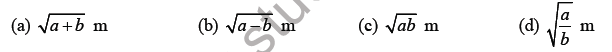Question : The angles of elevation of the top of a tower from two points at a distance of ‘a’ m and ‘b’ m from the base of the tower and in the same straight line with it are complementary, then the height of the tower isQuestion : From the top of a cliff 25m high the angle of elevation of a tower is found to be equal to the angle of depression of the foot of the tower. The height of the tower is
(a) 25 m
(b) 50 m
(c) 75 m
(d) 100 m

Question : If the angle of elevation of a cloud from a point 200m above a lake is 300 and the angle of depression of its reflection in the lake is 600, then the height of the cloud above the lake is
(a) 200 m
(b) 500 m
(c) 30 m
(d) 400 m

Question : The angle of elevation of a cloud from a point ‘h’ meter above a lake is ‘α’. The angle of depression of its reflection in the lake is 450. The height of the cloud is## Books recommended by teachers

 CBSE Class 10 Mathematics Real Numbers MCQs
 CBSE Class 10 Mathematics Polynomials MCQs
 CBSE Class 10 Mathematics Pairs of Linear Equations in Two Variables MCQs
 CBSE Class 10 Mathematics Quadratic Equations MCQs
 CBSE Class 10 Mathematics Arithmetic Progression MCQs
 CBSE Class 10 Mathematics Triangles MCQs
 CBSE Class 10 Mathematics Coordinate Geometry MCQs
 CBSE Class 10 Mathematics Introduction to Trigonometry MCQs
 CBSE Class 10 Mathematics Application of Trigonometry MCQs
 CBSE Class 10 Mathematics Circles MCQs
 CBSE Class 10 Mathematics Constructions MCQs
 CBSE Class 10 Mathematics Areas related to circles MCQs
 CBSE Class 10 Mathematics Surface Areas and Volumes MCQs
 CBSE Class 10 Mathematics Statistics MCQs
 CBSE Class 10 Mathematics Probability MCQs
 CBSE Class 10 Mathematics MCQs